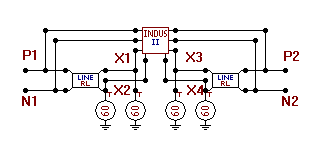﻿ MODELS > Examples > Models Other

# Models - Other Examples

1. Lightning induced voltage calculation

In this example a model for induced voltage calculations in overhead lines is presented. The example is taken from [1, 2]. The basic assumptions are:

The overhead line can be treated as loss-less.

The electrical field is assumed to propagate unaffected by the ground.

The Agrawal coupling model is used.

The transmission line (TL) model is used for the lightning channel, assuming a pure step current at ground. The actual current shape at ground is taken into account by a final convolution integral of the induced voltages. This assumption is believed to be valid the first few microseconds where often the maximum induced voltage occurs.

The electrical field from the lightning leader is constant, and the resulting induced voltage is zero.

The same inducing voltage is assumed in all phases, but this could easily be extended since the inducing voltage is proportional to the line height, z.

The circuit shown in Fig. 1 shows the equivalent circuit of and overhead line excited inducing fields from a lightning channel. It can be connected to any component in the ATP, and several line segments are allowed.Fig. 1. Equivalent circuit of 2-phase overhead line, excited by nearby lightning. Modeled in ATPDraw.

Below, an example of the electric circuit part of the two-phase model in Fig. 1 is listed in ATP file format.

/BRANCH

51X1    P1                  300.

52X2    N1                  200.             500.

51P2    X3                  300.

52N2    X4                  200.             500.

/SOURCE

60X1

60X2

60X3

60X4

A further simplification of this model is to rewrite it into a type94 Norton-transmission component.

References

 H.K. Høidalen, Lightning induced voltages in low-voltage systems, PhD thesis, ISBN 82-471-0177-1, University of Trondheim 1997.

 H.K. Høidalen, "Calculation of Lightning-induced Overvoltages using MODELS ", Proc. int. conf on Power Syst. Transients IPST'99, June 20-24, Budapest.

MODEL indloss2             --Calculation of lightning induced voltage in multi-phase overhead line with lossy ground effect

CONST Tmax  {VAL:500}     --number of time steps

n     {VAL:2}       --number of phases

c     {VAL:3.e8}     --speed of light

I0    {VAL:1}       --step current ampl.

eps0  {val:8.85e-12} --permittivity

sigma {VAL:0.001}   --ground conductivity

epsr  {VAL:10}       --relative permittivity

tc    {VAL:2e-6}     --current time to crest

th    {VAL:5e-5}     --current time to half value

v     {VAL:1.5e8}   --current velocity

INPUT UA[1..n],UB[1..n]   --terminal voltages

DATA XA0,YA0,XB0,YB0       --line position parameters (in meters in a global co-ordinate system)

z[1..n] {DFLT:10}     --phase heights

Im {DFLT:3e4}         --current amplitude

X0 {DFLT:0.0}         --lightning x-pos (in meters in a global co-ordinate system)

Y0 {DFLT:0.0}         --lightning y-pos

OUTPUT UrA[1..n],UrB[1..n] --type 60 sources

g1[0..Tmax], g2[0..Tmax],UrA[1..n],UrB[1..n],

Tr,i,AB,dt,uk[1..4],b,L,x,Ui0,UiD,tau,a1,b1,c0,c1,c2,c3,Y,XA,XB,a

FUNCTION F0(x,tr):= (c*tr-x)/(y*y+(b*(c*tr-x))**2)

FUNCTION F1(x,tr):= (x+b*b*(c*tr-x))/sqrt((v*tr)**2+(1-b*b)*(x*x+y*y))

FUNCTION F2(x,tr):= x+b*b*(c*tr-x)+sqrt((v*tr)**2+(1-b*b)*(x*x+y*y))

FUNCTION F3(x,tr):= (v*tr+sqrt((v*tr)**2+(1-b*b)*(x*x+y*y)))/sqrt(x*x+y*y)

FUNCTION t0(x):= sqrt(x*x+y*y)/c

HISTORY

UrA[1..n] {dflt:0}, UrB[1..n] {dflt:0}

UA[1..n]  {dflt:0}, UB[1..n]  {dflt:0}

DELAY CELLS (UA[1..n],UB[1..n],UrA[1..n],UrB[1..n]): (sqrt((XA0-XB0)**2+(YA0-YB0)**2))/(c*timestep)+1

INIT

a:=atan2(YA0-YB0,XA0-XB0)

XA:=(XA0-X0)*cos(a)+(YA0-Y0)*sin(a)

XB:=(XB0-X0)*cos(a)+(YB0-Y0)*sin(a)

Y :=(YA0-Y0)*cos(a)-(XA0-X0)*sin(a)

dt:= timestep

b:=v/c

L:=XA-XB

tau:=L/c

a1:=Im/(I0*tc)

b1:=0.5*tc/(th-tc)+1

c0:=PI*sigma/(eps0*epsr)

c1:=a1/sqrt(epsr)

c2:=-1.0736/c0+0.2153/(dt*c0**2)+4/3*sqrt(dt/c0)

c3:=-0.2153/(dt*c0**2)+1/6*sqrt(dt/c0)

FOR AB:=1 TO 2 DO

if AB=1 then

x:=XA else

x:=-XB

endif

FOR i:=0 TO Tmax DO

Tr:=i*dt

if Tr>t0(x)

then

if Tr>t0(x-L)+tau

then

Ui0:=f0(x,Tr)*(f1(x,Tr)-f1(x-L,Tr-tau))

UiD:=ln( f2(x,Tr)/f2(x-L,Tr-tau) )

-1/b*ln( f3(x,Tr)/f3(x-L,Tr-tau) )

else

Ui0:=f0(x,Tr)*(f1(x,Tr)+1)

UiD:=ln( f2(x,Tr)*f0(x,Tr) )

-1/b*ln( f3(x,Tr)/(1+b) )

endif

else

Ui0:=0

UiD:=0

endif

if AB=1 then

UindA0[i]:=Ui0

g1[i]:=a1*dt

if i>0

then

g2[i]:=a1*sqrt(eps0/(PI*sigma*Tr))*dt

else

g2:=c1*dt

endif

else

UindB0[i]:=Ui0

UindBD[i]:=UiD

endif

ENDFOR

ENDFOR

i:=Tmax

WHILE i>1 DO

uk[1..4]:=0

FOR Tr:=1 TO i-1 DO

uk:=uk+UindA0[Tr]*g1[i-Tr]

uk:=uk+UindB0[Tr]*g1[i-Tr]

uk:=uk+UindBD[Tr]*g2[i-Tr]

ENDFOR

UindA0[i]:=uk+0.5*UindA0[i]*g1

UindB0[i]:=uk+0.5*UindB0[i]*g1

UindBD[i]:=uk+(UindBD[i]*c2+UindBD[i-1]*c3)*c1

--UindBD[i]:=uk+0.5*UindBD[i]*g2

--UindBD[i]:=uk-0.5*UindBD[i-1]*g2

i:=i-1

ENDWHILE

Tr:=trunc(tc/dt)

FOR i:=Tmax TO Tr BY -1 DO

UindA0[i]:=UindA0[i]-b1*UindA0[i-Tr]

UindB0[i]:=UindB0[i]-b1*UindB0[i-Tr]

UindBD[i]:=UindBD[i]-b1*UindBD[i-Tr]

ENDFOR

ENDINIT

EXEC

FOR i:=1 to n DO

UrA[i]:=60*I0*b*( z[i]*UindA0[t/dt] - UindAD[t/dt] ) +

2*delay(UB[i],tau-dt,1)-delay(UrB[i],tau,1)

UrB[i]:=60*I0*b*( z[i]*UindB0[t/dt] - UindBD[t/dt] ) +

2*delay(UA[i],tau-dt,1)-delay(UrA[i],tau,1)

ENDFOR

ENDEXEC

ENDMODEL

2. Phasor-calculation, initialized from ATP's steady state

The example illustrates how the timestep can be changed internally to handle down-sampling, and how the steady-state values of the signal is used.

MODEL ABC2PHRI

comment---------------------------------------------------------------------

The model calculates the phasor of the input voltage with initial conditions.

Downsampling and algorithm selection.

------------------------------------------------------------------endcomment

INPUT

X[1..3]       --Input signal to be transformed

Xim[1..3]     --Imaginary part of steady-state signal (real part is X)

DATA

FREQ {DFLT:50}       --Power frequency

SampleFreq {DFLT:400} --Sample frequency S/s

Algorithm {dflt:0}   --0:FFT Radix 2-8, 1: DFT recursive

OUTPUT

reX[1..3],imX[1..3]   --Phasor

VAR

reX[1..3], imX[1..3],j,OMEGA,NSAMPL,D,alpha,x1,x3,x5,x7,xR,xI,delta_T[0..7]

TIMESTEP min: recip(SampleFreq)

DELAY CELLS (X[1..3]): recip(FREQ*timestep)+2

INIT

OMEGA:= 2*PI*FREQ

NSAMPL:=recip(FREQ*timestep)

for j:=1 to 3 do

reX[j]:=X[j]

imX[j]:=Xim[j]

endfor

alpha:=1/sqrt(2)

for j:= 0 to 7 do

delta_T[j] := j/(FREQ*8)

endfor

ENDINIT

HISTORY

X {DFLT:sqrt(reX**2+imX**2)*cos(t*omega+atan2(imX,reX))}

X {DFLT:sqrt(reX**2+imX**2)*cos(t*omega+atan2(imx,reX))}

X {DFLT:sqrt(reX**2+imX**2)*cos(t*omega+atan2(imX,reX))}

EXEC

for j:=1 to 3 do

if Algorithm=0

then

x1 := delay(X[j],delta_T,2) - delay(X[j],delta_T,2)

x3 := delay(X[j],delta_T,2) - delay(X[j],delta_T,2)

x5 := delay(X[j],delta_T,2) - delay(X[j],delta_T,2)

x7 := delay(X[j],delta_T,2) - delay(X[j],delta_T,2)

xR := x1 + (x5 - x7)*alpha

xI := x3 + (x5 + x7)*alpha

reX[j] := (xR*cos(OMEGA*T)+xI*sin(OMEGA*T))/4

imX[j] := (xI*cos(OMEGA*T)-xR*sin(OMEGA*T))/4

else

x1:=delay(X[j],1/FREQ,2)

D:=2/NSAMPL*(X[j]-x1)*cos(OMEGA*T)

reX[j]:=reX[j]+D

D:=2/NSAMPL*(X[j]-x1)*sin(OMEGA*T)

imX[j]:=imX[j]-D

endif

endfor

ENDEXEC

ENDMODEL